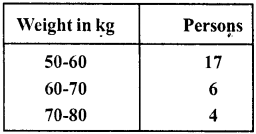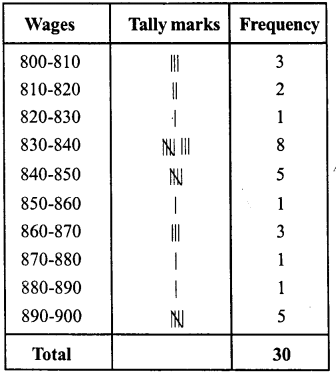# RD Sharma Class 8 Solutions Chapter 23 Data Handling I Ex 23.2

In this chapter, we provide RD Sharma Class 8 Solutions Chapter 23 Data Handling Ex 23.2 for English medium students, Which will very helpful for every student in their exams. Students can download the latest RD Sharma Class 8 Solutions Chapter 23 Data Handling Ex 23.2 pdf, free RD Sharma Class 8 Solutions Chapter 23 Data Handling Ex 23.2 book pdf download. Now you will get step by step solution to each question.

### RD Sharma Class 8 Solutions Chapter 23 Data Handling Ex 23.2Download PDF

Question 1.
The marks obtained by 40 students of class VIII in an examination are given below :
16, 17, 18, 3, 7, 23, 18, 13, 10, 21, 7, 1, 13,
21, 13, 15, 19, 24, 16, 3, 23, 5, 12, 18, 8, 12, 6,
8, 16, 5, 3, 5, 0, 7, 9, 12, 20, 10, 2, 23.
Divide the data into five groups, namely 0-5,5-10,10-15,15-20 and 20-25 and prepare a grouped frequency table.
Solution:
Frequency distribution tableQuestion 2.
The marks scored by 20 students in a test are given below :
54, 42, 68, 56, 62, 71, 78, 51, 72, 53, 44, 58, 47, 64, 41, 57, 89, 53, 84, 57.
Complete the following frequency table :What is the class interval in which the greatest frequency occurs ?
Solution:The class in which the greatest frequency is 50-60

Question 3.
The following is the distribution of weights (in kg) of 52 persons :(i) What is the lower limit of class 50-60 ?
(ii) Find the class marks of the classes 40-50, 50-60.
(iii) What is the class size ?
Solution:
(i) Lower limit of class 50-60 = 50
(ii) Class marks of 40-50 = 40+502 = 902
= 45 and of 50-60 = 50+602 = 1102 =55
(iii) Class size is 10

Question 4.
Construct a frequency table for the following weights (in gm) of 35 mangoes using the equal class intervals, one of them is 40-45 (45 not included):
30,40,45,32,43,50,55,62,70,70,61,62, 53,52, 50,42,35,37,53,55,65,70, 73, 74,45, 46, 58, 59, 60, 62, 74, 34, 35, 70 ,68.
(i) What is the class mark of the class interval 40-45 ?
(ii) What is the range of the above weights ?
(iii) How many classes are there ?
Solution:
Smallest observation = 30
Greatest observation = 74
Range = 74 – 30 = 44
Now forming the distribution table(i) Class mark of 40-45
= 40+452 = 852 = 42.5
(ii) Range = 74 – 30 = 44
(iii) Number of classes are 9

Question 5.
Construct a frequency table with class-intervals 0-5 (5 not included) of the following marks obtained by a group of 30 students in an examination :
0, 5, 7,10, 12,15, 20, 22, 25, 27, 8, 11, 17,3, 6, 9,17,19, 21, 29, 31,35,37,40,42, 45, 49, 4, 50, 16.
Solution:
Frequency distribution table.Question 6.
The marks scored by 40 students of class VIII in mathematics are given below:
81,55, 68, 79,85,43,29,68,54,73,47, 35, 72,64,95,44,50, 77,64,35,79, 52, 45,54,70,83, 62′, 64,72,92,84,76,63, 43, 54, 38, 73, 68, 52, 54.
Prepare a frequency distribution with class size of 10 marks.
Solution:
Largest marks = 95
Lowest marks = 29
Range = 95 – 29 = 66
Frequency distribution tableQuestion 7.
The heights (in cm) of 30 students of class VIII are given below :
155.158.154.158.160.148.149.150.153, 159,161,148,157,153,157,162,159,151, 154,156,152,156,160,152,147,155,163,155,157,153.
Prepare a frequency distribution table with 160-164 as one of the class intervals.
Solution:
Largest height =163
Lowest height =147
Range = 163- 147 = 16Question 8.
The monthly wages of 30 workers in a factory are given below :
830,835,890,810,835,836,869,845,898, 890, 820, 860, 832, 833, 855, 845, 804, 808, 812, 840, 885, 835, 836, 878, 840, 868, 890, 806, 840, 890.
Represent the data in the form of a frequency distribution with class size 10.
Solution:
Highest wage = 898
Lowest wage = 804
Range = 898 – 804 = 94
Frequency distribution tableQuestion 9.
Construct a frequency table with equal class intervals from the following data on the monthly wages (in rupees) at 28 labourers working in a factory, taking one of the class intervals as 210-230 (230 not included):
220, 268, 258, 242, 210, 268, 272,242, 311, 290, 300, 320,319,304,302,318,306,292, 254, 278, 210,240, 280,316,306, 215, 256, 236.
Solution:
Highest wages = 320
Lowest wages = 210
Range = 320-210= 110
Frequency distribution tableQuestion 10.
The daily minimum temperatures in degrees Celsius recorded in a certain Arctic region are as follows :
-12.5, -10.8, -18.6, -8.4, -10.8, -4.2, -4.8, -6.7, -13.2, -11.8, -2.3,1.2, 2.6, 0, -2.4, 0, 3.2, 2.7,3.4,0, -2.4, -2.4, 0,3.2, 2.7,3.4, 0,2.4, -5.8, -8.9, -14.6, -12.3, -11.5, -7.8, – 2.9
Represent them as frequency distribution table taking -19.9 to -15 as the first class interval.
Solution:
Lowest temperature = -19.9
Highest temperature = 3.4
Frequency distribution tableAll Chapter RD Sharma Solutions For Class 8 Maths

—————————————————————————–

All Subject NCERT Exemplar Problems Solutions For Class 8

All Subject NCERT Solutions For Class 8

*************************************************

I think you got complete solutions for this chapter. If You have any queries regarding this chapter, please comment on the below section our subject teacher will answer you. We tried our best to give complete solutions so you got good marks in your exam.

If these solutions have helped you, you can also share rdsharmasolutions.in to your friends.